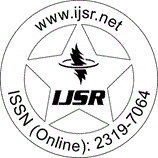International Journal of Science and Research (IJSR)
Call for Papers | Fully Refereed | Open Access | Double Blind Peer Reviewed

Research Paper | Mathematics | India | Volume 3 Issue 12, December 2014

# Integral Solutions of the Homogeneous Biquadratic Diophantine Equation with 6 Unknowns(x)^3-y^3)Z=(W^2-P^2)R^2

Dr. P. Jayakumar  | K. Sangeetha 

Abstract: The homogeneous biquadratic equation with 6 unknowns represented by (x^3-y^3) Z= (W^2-P^2) R^2 is analyzed for its non - zero distinct integer solutions. Employing the transformations and applying the method of factorization, five different patterns of non-zero distinct integer solutions to the above biquadratic equation are obtained. A few interesting relations between the solutions and special numbers namely Polygonal numbers and Pyramidal numbers are presented.

Keywords: Biquadratic equation with 6 unknowns, Homogeneous Biquadratic equation, integral solutions, Special numbers, A few interesting relations

Edition: Volume 3 Issue 12, December 2014,

Pages: 1021 - 1023